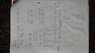# Derivatives of a higher order - Satisfying the equation

• K.QMUL

## Homework Statement

Show that y= xex satisfi es

A(d2y)/dx2 + B(dy/dx) + Cy = 0

for suitably chosen values of the constants A, B, and C.

Y=xex

## The Attempt at a Solution

Please see the attachment. I get to a point where I need to find the value of A, B and C but cannot as I'm dealing with x2 terms.

Help would be much appreciated.

#### Attachments

•20131015_172043.jpg
31.5 KB · Views: 413
You calculated dv/dx wrong.

Could you explain Where I went wrong?

Oh, I see the mistake, Thanks